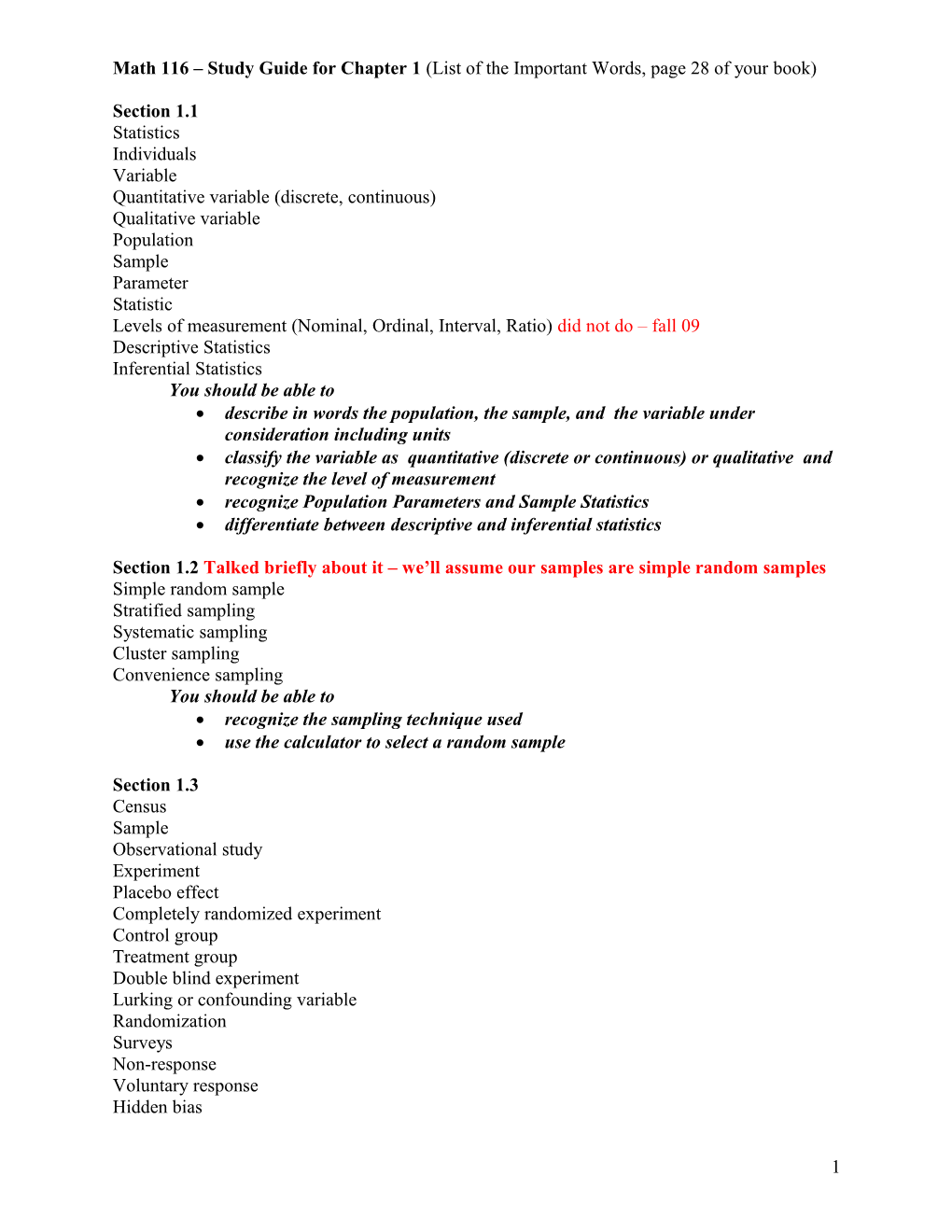Math 116 Study Guide for Chapter 1 (List of the Important Words, Page 28 of Your Book)Section 1.1

Statistics

Individuals

Variable

Quantitative variable (discrete, continuous)

Qualitative variable

Population

Sample

Parameter

Statistic

Levels of measurement (Nominal, Ordinal, Interval, Ratio)did not do – fall 09

Descriptive Statistics

Inferential Statistics

You should be able to

• describe in words the population, the sample, and the variable under consideration including units
• classify the variable as quantitative (discrete or continuous) or qualitative and recognize the level of measurement
• recognize Population Parameters and Sample Statistics
• differentiate between descriptive and inferential statistics

Section 1.2Talked briefly about it – we’ll assume our samples are simple random samples

Simple random sample

Stratified sampling

Systematic sampling

Cluster sampling

Convenience sampling

You should be able to

• recognize the sampling technique used
• use the calculator to select a random sample

Section 1.3

Census

Sample

Observational study

Experiment

Placebo effect

Completely randomized experiment

Control group

Treatment group

Double blind experiment

Lurking or confounding variable

Randomization

Surveys

Non-response

Voluntary response

Hidden bias

You should be able to

• distinguish between observational study and experiment

As you are solving problems throughout the semester, always think about all of the following:

• Describe the population in words
• Describe the variable including units
• Classify the variable as qualitative or quantitative
• Classify quantitative variables as discrete or continuous
• Describe the level of measurementdid not do – fall 09
• Is it an observational study or an experiment?
• Is this an example of descriptive or inferential statistic?
• What sampling technique could have been used?did not stress that much – fall 09
• Are you dealing with a sample or a population?
• Are the numbers that describe the data a statistic or a parameter?

Section 2.1

Frequency table

Classes, frequencies, relative frequency, cumulative frequency

Lower and upper limits of a class

Class boundaries

Midpoints

Class width

Computing the class width from (i) RAW DATA, (ii) GROUPED DATA

Constructing frequency table

Histograms

Shapes of distributions

(mound shaped symmetrical, uniform or rectangular, skewed to the left or right, bimodal)

Cumulative Frequency tables

Ogives

Frequency polygons

Dot plots

You should be able to

• recognize and sketch each of the mentioned graphs
• find the class width of a frequency table

a) Given a frequency table

b) Given raw data (a list of numbers)

• construct a frequency table by hand
• use the calculator to sketch a histogram
• use the histogram to construct the frequency table
• recognize shapes of distributions

Section 2.2

Bar graphs

Pareto charts

Circle graphs (computing the central angle)

Time-series graphs

You should be able to

• recognize and sketch each of the mentioned graphs

Section 2.3

EDA (Exploratory Data Analysis)

Stem and leaf plots

Split stem and leaf plots

Back to back stem plots

You should be able to

• recognize and sketch each of the mentioned graphs

Math 116 – Study Guide for Chapter 3 (List of the Important Words, page 148 of your book)

Section 3.1 – Measures of central tendency

Average

Mean

Notations for samples and for populations

Median

Mode

Resistant measure

Trimmed mean

Computing the 5% (or 10%) trimmed mean

Weighted mean or average

You should be able to

• recognize and use the notation for samples and populations
• compute the mean, median and mode by hand
• compute the mean with the calculator
• compute the trimmed mean
• compute the weighted mean

Section 3.2 – Measures of variation

Range

Standard deviation

Notations for samples and for populations

Variance

Rounding rules

Coefficient of variation(Did not do – fall 09)

Chebyshev’s theorem

Empirical rule (from section 6.1)

Range rule of thumb

Computing intervals about the mean in which at least ___% fall, according to the Empirical rule or Chebyshev’s theorem

Mean of grouped data

Standard deviation of grouped data

You should be able to

• recognize and use the notation for samples and populations
• compute the variance and standard deviation by hand and with the calculator
• compute the coefficient of variation
• use and interpret the Chebyshev’s theorem
• use and interpret the Empirical rule
• use the standard deviation as a ruler and identify usual and unusual values according to the range rule of thumb
• use the calculator to find the mean and standard deviation of grouped data

Section 3.3

Percentiles

Quartiles

Interquartile range, IQR

Five-number summary

Outliers

Box and whisker plots

Finding the lower and upper fences in order to find outliers(did not do – fall 09)

You should be able to

• find percentiles, and quartiles by hand and with the calculator
• find the five-number summary by hand and with the calculator
• find the interquartile range by hand
• construct box plots by hand
• find the fences that help in finding outliers
• FALL O9 – THIS IS WHAT I EXPECT THAT YOU KNOW:
• Construct box plots with the calculator and give the five-number-summary
• Identify the outliers and the smallest and largest numbers that are not outliers
• Identify shapes of distributions based on the box plots
• Compare box plots and give conclusions about the data based on the box plots
• Understand what the interquartile tells you
• We did not find percentiles other than the quartiles. In chapter 6 we’ll find percentiles

1